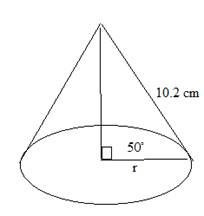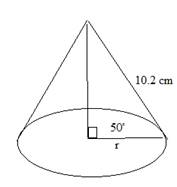Chapter 11.2, Problem 38E### Elementary Geometry for College St...

6th Edition
Daniel C. Alexander + 1 other
ISBN: 9781285195698

#### Solutions

Chapter
Section### Elementary Geometry for College St...

6th Edition
Daniel C. Alexander + 1 other
ISBN: 9781285195698
Textbook Problem
1 views

# In the right circular cone,a) Find r correct to tenths.b) Use L = π r l to find the lateral area of the cone.To determine

a) To find:

To find r correct to tenths by using the following figure,Explanation

Consider the following figure,

General formula for cosine ratio and sin ratio is given below,

From the above figure shows that 10

To determine

b) To find:

Use L=πrl to find the lateral area of the cone by using the following figure,### Still sussing out bartleby?

Check out a sample textbook solution.

See a sample solution

#### The Solution to Your Study Problems

Bartleby provides explanations to thousands of textbook problems written by our experts, many with advanced degrees!

Get Started

#### Evaluate the limit, if it exists. limt0(1t1t2+t)

Single Variable Calculus: Early Transcendentals, Volume I

#### Convert the expressions in Exercises 6584 to power form. 3x453x+43xx

Finite Mathematics and Applied Calculus (MindTap Course List)

#### Use a Table of Integrals to evaluate .

Study Guide for Stewart's Single Variable Calculus: Early Transcendentals, 8th